Courses

# Test: Soil Mechanics

## 20 Questions MCQ Test Mock Test Series of SSC JE Civil Engineering | Test: Soil Mechanics

Description
This mock test of Test: Soil Mechanics for SSC helps you for every SSC entrance exam. This contains 20 Multiple Choice Questions for SSC Test: Soil Mechanics (mcq) to study with solutions a complete question bank. The solved questions answers in this Test: Soil Mechanics quiz give you a good mix of easy questions and tough questions. SSC students definitely take this Test: Soil Mechanics exercise for a better result in the exam. You can find other Test: Soil Mechanics extra questions, long questions & short questions for SSC on EduRev as well by searching above.
QUESTION: 1

### In Boussinesq’s solution to the problem of stress distribution, all the following assumptions are made, except:

Solution:

Assumptions made in Boussinesq’s Theory are:

1. Soil is homogeneous, semi-infinite, elastic and isotropic.

2. Hooke’s law is valid.

3. Any change in the volume of soil due to application of load is neglected.

4. Distribution of stresses along the vertical axis is symmetrical.

5. Self-weight of soil is neglected.

QUESTION: 2

### Spring analogy in soil mechanics is used for explaining which of the following phenomenon?

Solution:

Terzaghi in 1922 demonstrated a model (Spring-piston model) to study the mechanism of consolidation in clay. The model consists of a cylindrical vessel with the series of pistons separated by springs. The space between the springs is filled with water and the pistons are perforated so as to allow through communication to water. Piezometers are inserted at the centres of each of the compartments to measure the pressure heads due to excess pore water pressures. The springs surrounded by water represent the saturated soil. The springs represents the soil skeleton and the water in the vessel represents the water filling the voids in the soil.

QUESTION: 3

### The term _________ on soil is used to indicate the maximum pressure which can be exerted on soil while taking into account shear failure, settlement and the ability of structure to resist settlement.

Solution:

The value of Allowable Bearing Pressure on soil depends on the nature of ground and the type of building. The maximum safe bearing capacity of soil is generally more than the allowable bearing pressure on soil.

QUESTION: 4

If the coefficient of uniformity of a soil is 16 and the coefficient of curvature is 1, then what is the ratio D60/D30?

Solution:

Cu = 16

Cc = 1

16=Cu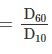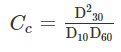D60 = 16 D10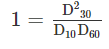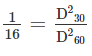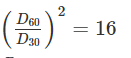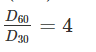QUESTION: 5

The shear test in which full consolidation occurs and excess pore pressure is build up during shearing stage is known as:

Solution:

In first stage (application of cell pressure stage) soil will consolidate because drainage valve is open and pore water is drain out of soil leads to consolidation, In second stage (Shearing stage) drainage valves are closed leads to development of excess pore water pressure, this implies first stage is consolidated(C) and second stage is undrained(U). So the test is CU test.

QUESTION: 6

The permeability of a soil varies:

Solution: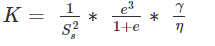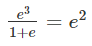Where Ss = specific surface area =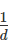Where d = grain size

So, permeability is directly proportional to the square of grain size.

QUESTION: 7

A soil mass has a void ratio of 0.67? How much water (in litres) will be required to fully saturate 1 m3 of the soil?

Solution:

e = 0.68

Volume of soil = 1m3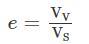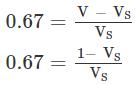0.67 VS = 1 – VS

1.67 VS = 1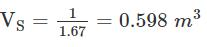VV = V – VS = 1 – 0.598 = 0.402 m3

Initially we assume that soil is completely dry

VV = Vwater required to fully saturate the soil mass

Vwater = 0.402 × 1000 = 402 litres

QUESTION: 8

If the fineness modulus of sand is 3, then the sand is graded as

Solution:

Fineness modulus of sand:

1. Fine sand: 2.2 to 2.6

2. Medium sand: 2.6 to 2.9

3. Coarse sand: 2.9 to 3.2

So, Fineness modulus 3 corresponds to coarse sand.

QUESTION: 9

Find the shrinkage ration of a soil sample whose plastic limit and liquid limit values are 30% and 42%, respectively, and the values of percentage of volume change from liquid limit to dry state and plastic limit to dry state are 35% and 22%, respectively, of dry volume.

Solution:

corresponding change in the water content of the soil above shrinkage limit.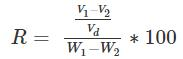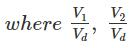are percentage change of volume from liquid limit to dry state and plastic limit to dry state respectively

W1 and W2 are corresponding water content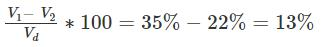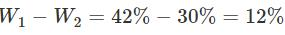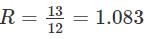QUESTION: 10

In vane shear test, the torque rod is rotated at a uniform speed of:

Solution:

In Vane shear test, the vane is pushed in to the soil and torque is applied manually which is measured by a calibrated torsional spring. The rate of rotation of vane is nearly 6per minute or 0.1o per second.

QUESTION: 11

Which of the following shows the CORRECT order of increasing surface areas of the given soil?

Solution:

Specific Surface area is inversely proportional to grain size. Out of sand, silt, clay and colloids, Sand has highest grain size while colloids have least grain size.

QUESTION: 12

What is the assumption made about back of wall, in the Rankine’s theory of earth pressure?

Solution:

Assumptions given by Rankine are:

1. The soil is homogeneous and isotropic, which means c, φ and γ have the same values everywhere, and they have the same values in all directions at every point.

2. The most critical shear surface is a plane.

3. The ground surface is a plane.

4. The wall is infinitely long so that the problem may be analysed in only two dimensions.

5. The wall moves sufficiently to develop the active or passive condition.

6. The wall surface at the back is vertical and smooth.

QUESTION: 13

If in a soil mass, the product of degree of saturation (s) and void ratio (e) is 1. Then water content (ω) is

Solution:

Se = ωG

1 = ωG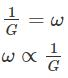QUESTION: 14

A soil has a bulk density of 22 kN/m3 and water content of 12%. The dry density of soil is

Solution: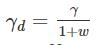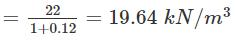QUESTION: 15

What is the ratio of discharge through the tube well to the discharge through the open well

Solution:

Discharge through the open well is in the range of (3-6) litres/sec while discharge through the tube well is in the range of (200 - 220) l/sec. So the approximate ratio is 50.

QUESTION: 16

The pile provided with one or more bulbs in its vertical shaft, is generally known as

Solution:

The pile provided with one or more bulbs in the vertical shaft is known as under reamed pile. This kind of pile is provided in case of expansive soil like black cotton soil.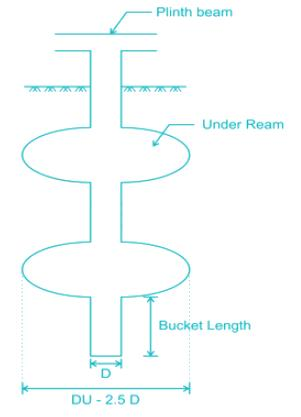QUESTION: 17

The angle of inclination of the plane at which the body begins to move down the plane, is called

Solution:

The angle of inclination of the plane at which the body begins to move down the plane, is called angle of repose.

QUESTION: 18

Match List I (Types of Soil) with List II (weathering agent) and select the answer option given below:-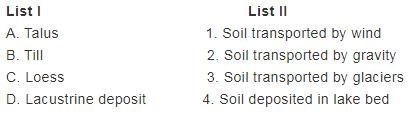Solution: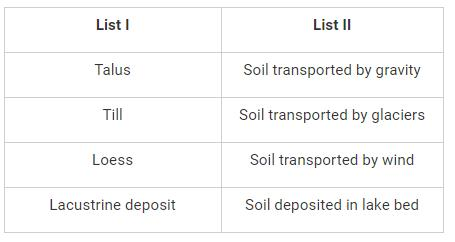QUESTION: 19

The maximum height of an unsupported vertical cut in a cohesive soil is given by ________

Solution:

Depth at which the earth pressure become zero in case of cohesive soil is given by expression,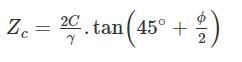So the depth of unsupported vertical cut is,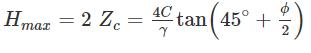QUESTION: 20

Shear strength of soil increases with increase in -

Solution:

Shearstrength,τ=C+σtanϕ

Where C = Cohesion

σ = Nomal stress

ϕ = Angle of internal friction.

So all the above options are correct. Shear strength will increase with increase in cohesion, angle of internal friction and normal stress on soil.